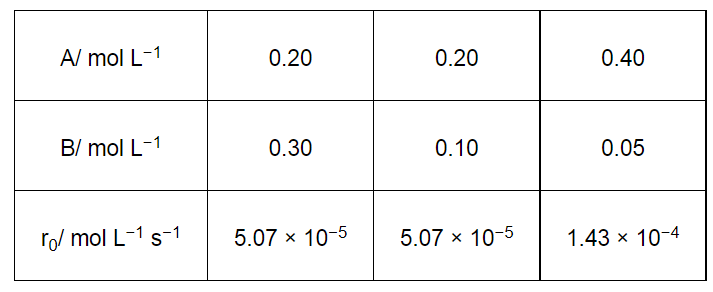# In a reaction between A and B,Question:

In a reaction between A and B, the initial rate of reaction (r0) was measured for different initial concentrations of A and B as given below:What is the order of the reaction with respect to A and B?

Solution:

Let the order of the reaction with respect to A be x and with respect to B be y.

Therefore,

$\mathrm{r}_{0}=k[\mathrm{~A}]^{x}[\mathrm{~B}]^{y}$

$5.07 \times 10^{-5}=k[0.20]^{x}[0.30]^{y}$         ...(i)

$5.07 \times 10^{-5}=k[0.20]^{x}[0.10]^{y}$          ...(ii)

$1.43 \times 10^{-4}=k[0.40]^{x}[0.05]^{y}$          ....(iii)

Dividing equation (i) by (ii), we obtain

$\frac{5.07 \times 10^{-5}}{5.07 \times 10^{-5}}=\frac{k[0.20]^{x}[0.30]^{y}}{k[0.20]^{x}[0.10]^{y}}$

$\Rightarrow 1=\frac{[0.30]^{y}}{[0.10]^{y}}$

$\Rightarrow\left(\frac{0.30}{0.10}\right)^{0}=\left(\frac{0.30}{0.10}\right)^{y}$

$\Rightarrow y=0$

Dividing equation (iii) by (ii), we obtain

$\frac{1.43 \times 10^{-4}}{5.07 \times 10^{-5}}=\frac{k[0.40]^{x}[0.05]^{y}}{k[0.20]^{x}[0.30]^{y}}$

$\Rightarrow \frac{1.43 \times 10^{-4}}{5.07 \times 10^{-5}}=\frac{[0.40]^{x}}{[0.20]^{x}} \quad\left[\begin{array}{l}\text { Since } y=0, \\ {[0.05]^{y}=[0.30]^{y}=1}\end{array}\right]$

$\Rightarrow 2.821=2^{x}$

$\Rightarrow \log 2.821=x \log 2$         (Taking log on both sides)

$\Rightarrow x=\frac{\log 2.821}{\log 2}$

= 1.496

= 1.5 (approximately)

Hence, the order of the reaction with respect to A is 1.5 and with respect to B is zero.